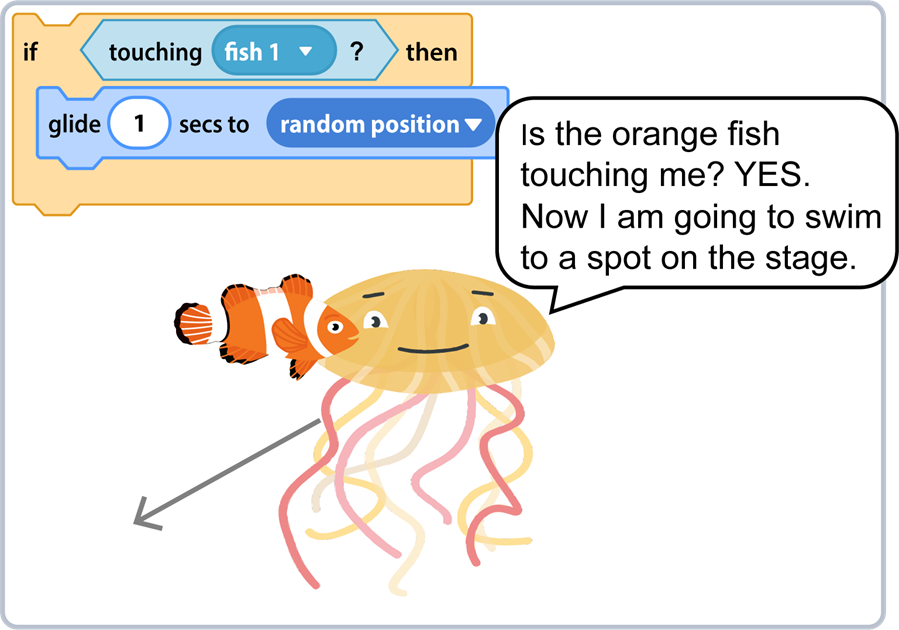# Teaching If Then Statements, Make Real World Connections

Make real world connections when teaching if then statements to beginners. If then statements are conditionals. A conditional is an action that occurs if something specific happens. If then statements are used in programming to trigger a set of instructions.

If then statements can be a difficult concept for young children to understand. To help them comprehend the logic, compare it to situations in their daily life. For instance, you may go home from school but only if it is the end of the day – then you can leave. Or you may get a treat, but only if you eat your dinner – then you can have a cookie.

## Teaching If Then Statements to Beginners

Think about a school day. IF an event occurs THEN what happens?

• if it is math class then ___
• if it is library day then ___
• if the fire alarm rings then ___
• if the teacher is talking then ___

Think about a sporting event. IF an event occurs THEN what happens?

• if the referee blows the whistle then ___
• if a time limit is reached then ___
• if a racer crosses the finish line first then ___
• if a player touches a friend during tag then ___
• if a soccer ball goes out of bounds then ___

Think about weather. IF an event occurs THEN what happens?

• if it is hot then wear ___
• if it is cold then wear ___
• if it is rainy then wear___

Think about your daily life. IF an event occurs THEN what happens?

• if the clock alarm goes off in the morning then ___
• if you do not clean your room then ___
• if the traffic light is red then ___

• if a cell phone battery charge is 0 then ___
• if a person forgets to sign out of a device then ___
• if the microwave reaches the time set for cooking then ___
• if the SHIFT key is pressed when typing a letter then ___
• if the Fill tool is clicked by the mouse in a drawing program then ___

## Teaching If Then Statements using Scratch

When teaching if then statements it is essential that students understand the logic FIRST – before programming begins. Once connections have been made to daily life, students are ready to build scripts with if then coding statements. The if then coding block in Scratch, a coding app for children, can be used to direct the action in animated scenes, puzzles, or games. It uses blocks that are joined together to form scripts. The if then coding block has a blank spot that is filled with the condition.

In Scratch the if then condition can be:

• if a sprite is touching another sprite
• if a sprite touches the mouse pointer
• if a sprite reaches the edge of the stage
• if a sprite touches a color
• if one color is touching another color
• if a keyboard key is pressed
• if the mouse button is pressed down
• if a value equals an amount
• if a value is less than an amount
• if a value is greater than an amount

## Lessons to Teach If Then Statements

Are you interested in teaching a coding unit that applies if then logic? TechnoCode uses if then statements to have students build scripts to control the action of characters. Several assignments use conditionals:

• Animated Fish Tank: Sense if a sprite is touching a specific fish. If it is, then alter movement. Children can program the sprite to swim to a new spot, change direction, or spin.
• Maze: Sense if a sprite touches a color outside the path. If it is, then move back to stay inside the path.
• Game: Sense if a player touches a target. If it does, then increase the score.
• Game: Determine if a final score in a game is greater than a value. If it is, then display a message such as “You won!”